FanDuel Championship Odds
+280
2.8 to 1Gonzaga
12.4% implied probability

+500
5 to 1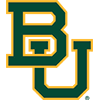Baylor
7.9% implied probability

+900
9 to 1Villanova
4.7% implied probability

+1100
11 to 1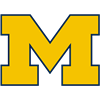Michigan
3.9% implied probability

+1300
13 to 1Iowa
3.4% implied probability

+1400
14 to 1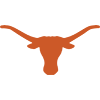Texas
3.2% implied probability

+1700
17 to 1Wisconsin
2.6% implied probability

+1700
17 to 1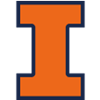Illinois
2.6% implied probability

+2000
20 to 1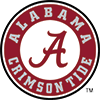Alabama
2.3% implied probability

+2000
20 to 1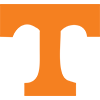Tennessee
2.3% implied probability

+2000
20 to 1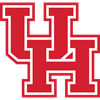Houston
2.3% implied probability

+2000
20 to 1Virginia
2.3% implied probability

+2200
22 to 1Creighton
2.1% implied probability

+2300
23 to 1West Virginia
2.0% implied probability

+2500
25 to 1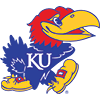Kansas
1.8% implied probability

+2500
25 to 1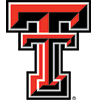Texas Tech
1.8% implied probability

+2700
27 to 1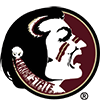Florida State
1.7% implied probability

+4000
40 to 1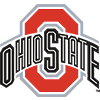Ohio State
1.2% implied probability

+4000
40 to 1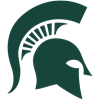Michigan State
1.2% implied probability

+4000
40 to 1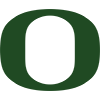Oregon
1.2% implied probability

+4500
45 to 1Duke
1.0% implied probability

+4500
45 to 1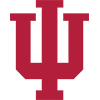Indiana
1.0% implied probability

+5000
50 to 1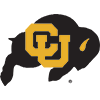Colorado
0.9% implied probability

+5000
50 to 1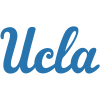UCLA
0.9% implied probability

+5000
50 to 1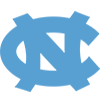North Carolina
0.9% implied probability

+5000
50 to 1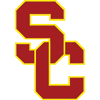USC
0.9% implied probability

+5000
50 to 1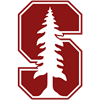Stanford
0.9% implied probability

+5500
55 to 1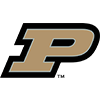Purdue
0.8% implied probability

+5500
55 to 1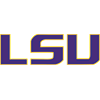Louisiana State
0.8% implied probability

+5500
55 to 1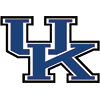Kentucky
0.8% implied probability

+5500
55 to 1Virginia Tech
0.8% implied probability

+5500
55 to 1Connecticut
0.8% implied probability

+6500
65 to 1Clemson
0.7% implied probability

+6500
65 to 1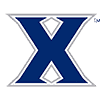Xavier
0.7% implied probability

+6500
65 to 1Saint Louis
0.7% implied probability

+6500
65 to 1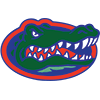Florida
0.7% implied probability

+6500
65 to 1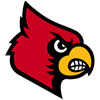Louisville
0.7% implied probability

+7500
75 to 1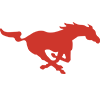SMU
0.6% implied probability

+7500
75 to 1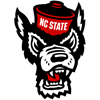North Carolina State
0.6% implied probability

+7500
75 to 1Missouri
0.6% implied probability

+7500
75 to 1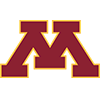Minnesota
0.6% implied probability

+7500
75 to 1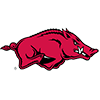Arkansas
0.6% implied probability

+7500
75 to 1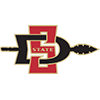San Diego State
0.6% implied probability

+8000
80 to 1Boise State
0.6% implied probability

+8000
80 to 1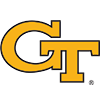Georgia Tech
0.6% implied probability

+8000
80 to 1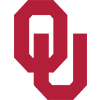Oklahoma
0.6% implied probability

+8500
85 to 1Richmond
0.5% implied probability

+8500
85 to 1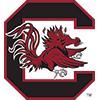South Carolina
0.5% implied probability

+8500
85 to 1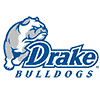Drake
0.5% implied probability

+8500
85 to 1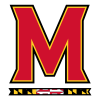Maryland
0.5% implied probability

+8500
85 to 1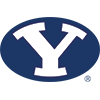BYU
0.5% implied probability

No changes have been recorded yet.
+8500
85 to 1Arizona State
0.5% implied probability

+10000
100 to 1Memphis
0.5% implied probability

+10000
100 to 1Dayton
0.5% implied probability

+10000
100 to 1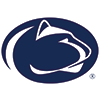Penn State
0.5% implied probability

+10000
100 to 1Providence
0.5% implied probability

+10000
100 to 1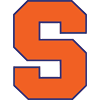Syracuse
0.5% implied probability

+10000
100 to 1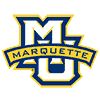Marquette
0.5% implied probability

No changes have been recorded yet.
+11000
110 to 1Pittsburgh
0.4% implied probability

+11000
110 to 1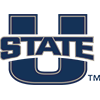Utah State
0.4% implied probability

+12000
120 to 1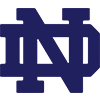Notre Dame
0.4% implied probability

+12000
120 to 1VCU
0.4% implied probability

No changes have been recorded yet.
+13000
130 to 1Butler
0.4% implied probability

+13000
130 to 1Wake Forest
0.4% implied probability

No changes have been recorded yet.
+15000
150 to 1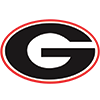Georgia
0.3% implied probability

No changes have been recorded yet.
+15000
150 to 1Utah
0.3% implied probability

+15000
150 to 1Davidson
0.3% implied probability

+15000
150 to 1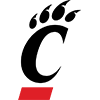Cincinnati
0.3% implied probability

+15000
150 to 1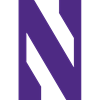Northwestern
0.3% implied probability

+15000
150 to 1Belmont
0.3% implied probability

No changes have been recorded yet.
+15000
150 to 1Wichita State
0.3% implied probability

+15000
150 to 1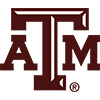Texas A&M
0.3% implied probability

No changes have been recorded yet.
+15000
150 to 1Temple
0.3% implied probability

+15000
150 to 1St. Mary's (CAL)
0.3% implied probability

+15000
150 to 1Western Kentucky
0.3% implied probability

+15000
150 to 1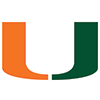Miami
0.3% implied probability

+15000
150 to 1Rhode Island
0.3% implied probability

+15000
150 to 1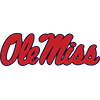Mississippi
0.3% implied probability

+15000
150 to 1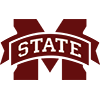Mississippi State
0.3% implied probability

+18000
180 to 1Wofford
0.3% implied probability

+20000
200 to 1DePaul
0.2% implied probability

+20000
200 to 1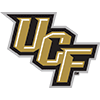Central Florida
0.2% implied probability

No changes have been recorded yet.
+20000
200 to 1Boston College
0.2% implied probability

No changes have been recorded yet.
+20000
200 to 1Nevada
0.2% implied probability

+20000
200 to 1UNLV
0.2% implied probability

+20000
200 to 1Washington State
0.2% implied probability

No changes have been recorded yet.
+20000
200 to 1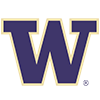Washington
0.2% implied probability

+20000
200 to 1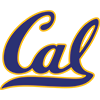California
0.2% implied probability

No changes have been recorded yet.
+20000
200 to 1Vanderbilt
0.2% implied probability

No changes have been recorded yet.
+20000
200 to 1Georgetown
0.2% implied probability

+20000
200 to 1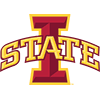Iowa State
0.2% implied probability

+20000
200 to 1Kansas State
0.2% implied probability

No changes have been recorded yet.
+20000
200 to 1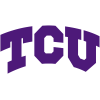TCU
0.2% implied probability

+20000
200 to 1Loyola-Chicago
0.2% implied probability

No changes have been recorded yet.
+20000
200 to 1St. John's
0.2% implied probability

+20000
200 to 1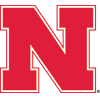Nebraska
0.2% implied probability

No changes have been recorded yet.
+20000
200 to 1Northern Iowa
0.2% implied probability